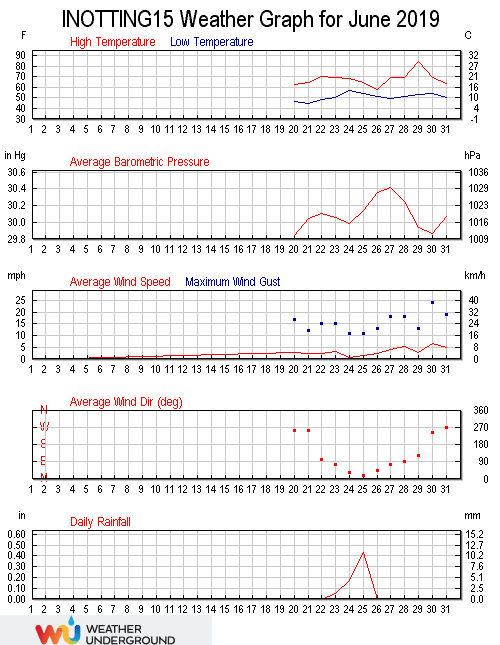June 2019 was cooler than average (13.7°C versus an average of 14.4), wetter than average (104.20 mm versus an average total of 52.56) and less windy than average (a wind run of 2240 miles versus an average of 2408).

The highest temperature in the month was 29.4°C, the highest temperature of 2019 so far, and the highest temperature since 31.0°C was recorded on July 26th 2018. This was the second highest June temperature recorded here, beaten only by the 29.5°C recorded on June 19th 2017. The minimum temperature was 7.0°C recorded at 05:03 on the 16th. The average temperature in the month was 13.7°C, 0.6°C below the June mean. The average daily high was 18.0°C, 0.7°C below the mean, with the average daily low being 9.8°C, 0.3°C below the mean. The warmest day was the 30th with an average temperature of 20.9, with the coolest day being the 11th with an average temperature of 9.4°C.

The average wind chill in the month was 13.6°C . The high dew point was 21.2°C at 16:48 on the 29th, with the low dew point being 4.7°C at 05:38 on the 7th. The average monthly dew point was 10.8°C. There were 185.0 hours of sunshine, with the sunniest day being the 22nd with 11.41 hours. The maximum heat index was 31.9°C at 17:58 on the 29th, a record high for June.

Rainfall in June was well above average with 104.2 millimetres falling on 18 days. This was well above the average of 57.4 millimetres. This was the wettest month since June 2012 when 127.8 millimetres was recorded, and the third wettest month ever recorded here. The year to date total is 275.2 millimetres, 106.7% of the average for the end of June. The wettest day was the 11th when 22.8 millimetres of rain fell, making it the wettest day since August 8th 2017 when 26.4 millimetres was recorded. The highest rain rate was 63.2 millimetres per hour at 00:00 on the 24th. There was no snow recorded.

The highest barometric pressure of 1031.7 millibars was at 09:41 on the 27th. The lowest pressure was 998.2 millibars at 19:38 on the 7th. The average pressure in the month was 1012.9 millibars. The highest humidity was 99% at 06:38 on the 12th, and the monthly average humidity was 83%. The lowest humidity was 48% at 17:16 on the 6th, a record low for June.

Winds were lower than average in the month, with the highest wind gust speed being 26 mph recorded at 18:22 on the 30th, 2.5 mph below the month average. The maximum wind speed was 14 mph at 18:55 on the 2nd, 2.6 mph below the mean. The wind run was 2239.7 miles, well below the June average of 2408.2 miles. The average gust speed in the month was 5.1 mph, 0.5 mph below the mean. The average wind speed was 3.2 mph, 0.1 mph below the mean. The predominant direction was south south-easterly (159°).

The maximum high solar radiation recorded at 13:33 on the 20th was 1176 w/m². The average solar radiation for the month was 163 w/m². The highest U.V. Index was 6.6 recorded at 11:58 on the 27th, the highest since 7.0 was recorded on August 3rd 2018.This site uses Akismet to reduce spam. Learn how your comment data is processed.